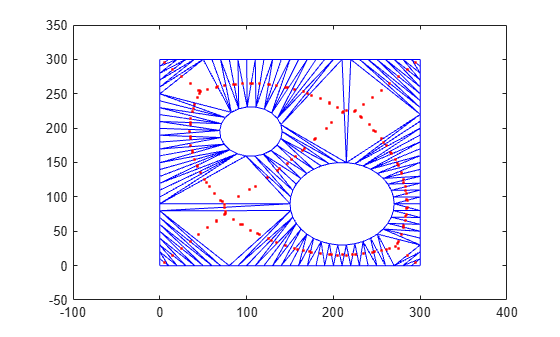# circumcenter

Circumcenter of triangle or tetrahedron

## Syntax

``C = circumcenter(TR)``
``C = circumcenter(TR,ID)``
``[C,r] = circumcenter(___)``

## Description

example

````C = circumcenter(TR)` returns the coordinates of the circumcenters for each triangle or tetrahedron in the triangulation `TR`.```

example

````C = circumcenter(TR,ID)` returns the coordinates of the circumcenters for the triangles or tetrahedra indexed by `ID`. The identification numbers of the triangles or tetrahedra in `TR` are the corresponding row numbers of the property `TR.ConnectivityList`.```

example

````[C,r] = circumcenter(___)` also returns the radii of the circumscribed circles or spheres in `TR` for either of the previous syntaxes.```

## Examples

collapse all

Load 2-D triangulation data and create a triangulation representation.

```load trimesh2d TR = triangulation(tri,x,y);```

Compute the circumcenters of each triangle in `TR`.

`C = circumcenter(TR);`

Plot the triangulation along with the circumcenters in red. The $x$-coordinates of the circumcenters are contained in the first column of `C` and the corresponding $y$-coordinates are contained in the second column.

```triplot(TR) axis([-100 400 -50 350]) hold on plot(C(:,1),C(:,2),'r.') hold off```Create a Delaunay triangulation for a set of points.

```rng default; P = rand(10,3); TR = delaunayTriangulation(P);```

Compute the circumcenters of the first five tetrahedra in `TR`, and the radii of their circumscribed spheres.

`[C,r] = circumcenter(TR,[1:5]')`
```C = 5×3 13.2189 -2.3004 -0.4970 -0.4884 0.6741 -0.1140 0.3089 0.1067 -0.3349 0.2805 0.7532 0.5855 0.8514 2.1609 0.9824 ```
```r = 5×1 12.6978 1.1775 1.1135 0.3670 1.4112 ```

## Input Arguments

collapse all

Triangulation representation, specified as a scalar `triangulation` or `delaunayTriangulation` object.

Data Types: `triangulation` | `delaunayTriangulation`

Triangle or tetrahedron identification, specified as a scalar or a column vector whose elements each correspond to a single triangle or tetrahedron in the triangulation object. The identification number of each triangle or tetrahedron is the corresponding row number of the `ConnectivityList` property.

Data Types: `double`

## Output Arguments

collapse all

Circumcenters of triangles or tetrahedra, returned as a two-column matrix for 2-D coordinates or a three-column matrix for 3-D coordinates.

Data Types: `double`

Radii of the circumscribed circles or spheres, returned as a scalar or vector.

Data Types: `double`